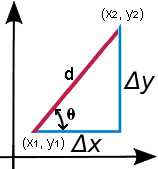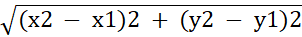# Slope Calculator

## Why use an online slope calculator?

With the help of slope calculator provide by Mycalcu you can easily find out the steepness, grade or incline of a line which is known as slope or gradient of a line.

No more slope find would be time consuming and difficult for you. Try this out since it's free of cost and there are no charges or any logging requirements at all.

## Slope Calculator Results

 Slope (m) Angle (Θ) Distance (d) ΔX ΔY

## How does it work?

Usually, a line's steepness is measured by the value of its slope, “m”. The larger this value would be, the steeper would be the line.

Mathematically the slope is represented as:

m =

 y2 - y1 x2 - x1

In the above equation, y2 - y1 = Δy represents vertical change, while x2 - x1 = Δx represents horizontal change.d = (x2 - x1)2 + (y2 - y1)2m = tan(θ)

By putting the values of x and y coordinates you will get your slope of the line

## How to use a slope calculator?

Enter the known coordinates or point which are known to you and then click calculate to get your solution in a jiffy.